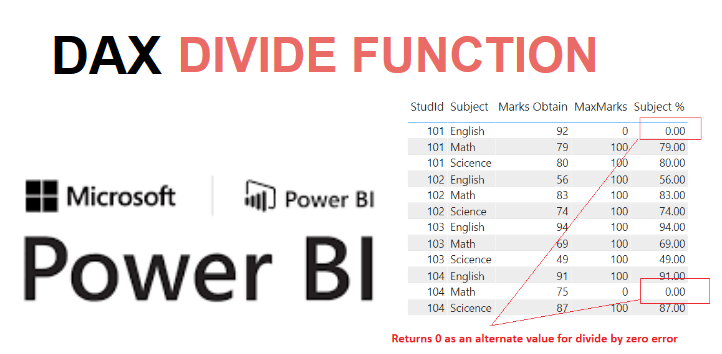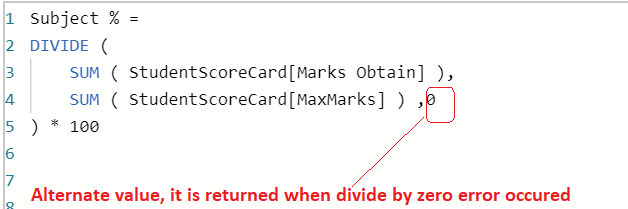Home » DIVIDE Function DAX

# DIVIDE Function DAX### DAX DIVIDE Function

DIVIDE function is a power bi DAX math and trig functions that performs the division and returns alternate result or BLANK() on division by 0.

It returns a decimal number.

SYNTAX

`DIVIDE(<numerator>, <denominator> [,<alternateresult>])`

numerator  The dividend or number to divide.

denominator  The divisor or number to divide by.

alternateresult  It is an optional, returned when division by zero error occurred.

By default it returns blank().

Lets look at an example of using DIVIDE function in Power Bi.

Here we have a sample dataset of student score card.

Note that, You can see MaxMarks is 0 for studid 101 , and 104 in Subject English and Math respectively.

Which are taken purposely to see the DIVIDE function ability to handle the divide by zero error, and return alternate value if it is given else blank.StudId           Subject               Marks Obtain   MaxMarks

 101 Math 79 100 101 Science 80 100 101 English 92 0 102 Math 83 100 102 Science 74 100 102 English 56 100 103 Math 69 100 103 Science 49 100 103 English 94 100 104 Math 75 0 104 Science 87 100 104 English 91 100

Lets say, you want to calculate the percentage achieved by student in each subject.

To calculate the percentage, first you need to divide Marks obtain (dividend) in subject by max marks (divisor) then multiple by the result by 100.

Lets use the DIVIDE function DAX for the division as given below.

```Subject % =

DIVIDE (

SUM ( StudentScoreCard[Marks Obtain] ),

SUM ( StudentScoreCard[MaxMarks] )

) * 100```After committing, the above DAX Subject %, Lets drag it into table visual as shown below.If you want to replace blank value with zero, then you can modify above DAX and provide an alternate value, when divide by zero error occurred.

```Subject % =

DIVIDE (

SUM ( StudentScoreCard[Marks Obtain] ),

SUM ( StudentScoreCard[MaxMarks] ) ,0

) * 100```As you can see, Blank value is replaced with an alternate value 0.This site uses Akismet to reduce spam. Learn how your comment data is processed.